# PointGravityWithPointMasses

Two point masses in a point gravity field (rotation of bodies is neglected)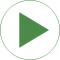# Information

This information is part of the Modelica Standard Library maintained by the Modelica Association.

This model demonstrates the usage of model Parts.PointMass in a point gravity field. The PointMass model has the feature that the rotation is not taken into account and can therefore also not be calculated. This example demonstrates two cases where this does not matter: If a PointMass is not connected (body1, body2), the orientation object in these point masses is set to a unit rotation. If a PointMass is connected by a line force element, such as the used Forces.LineForceWithMass component, then the orientation object is set to a unit rotation within the line force element. These are the two cases where the rotation is automatically set to a default value, when the physical system does not provide the equations.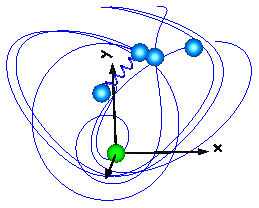# Components (6)

world body1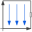Type: World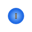Type: PointMassType: PointMassType: PointMassType: PointMass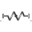Type: Spring